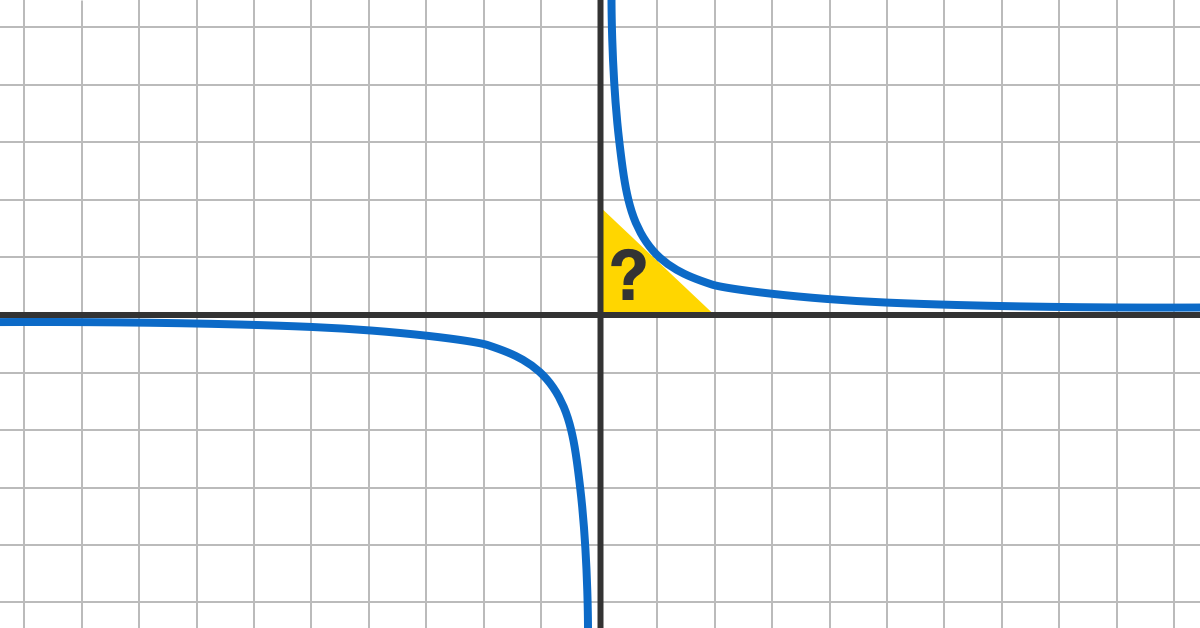# Tightly Bounded Area

Calculus Level 2A tangent to the function $f(x)=\dfrac{1}{x}$ is drawn on the first quadrant of the Cartesian plane.

Let $X$ denote the area bounded by this tangent, the $x$-axis and the $y$-axis.

What is the range of the values of $X$ can take?

×